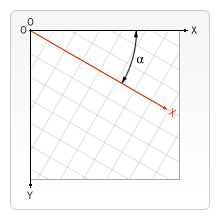# CanvasRenderingContext2D: rotate() method

The `CanvasRenderingContext2D.rotate()` method of the Canvas 2D API adds a rotation to the transformation matrix.

## Syntax

js

``````rotate(angle)
``````### Parameters

`angle`

The rotation angle, clockwise in radians. You can use `degree * Math.PI / 180` to calculate a radian from a degree.

The rotation center point is always the canvas origin. To change the center point, you will need to move the canvas by using the `translate()` method.

## Examples

### Rotating a shape

This example rotates a rectangle by 45°. Note that the center of rotation is the top-left corner of the canvas, and not a location relative to any shape.

#### HTML

html

``````<canvas id="canvas"></canvas>
``````

#### JavaScript

js

``````const canvas = document.getElementById("canvas");
const ctx = canvas.getContext("2d");

// Point of transform origin
ctx.arc(0, 0, 5, 0, 2 * Math.PI);
ctx.fillStyle = "blue";
ctx.fill();

// Non-rotated rectangle
ctx.fillStyle = "gray";
ctx.fillRect(100, 0, 80, 20);

// Rotated rectangle
ctx.rotate((45 * Math.PI) / 180);
ctx.fillStyle = "red";
ctx.fillRect(100, 0, 80, 20);

// Reset transformation matrix to the identity matrix
ctx.setTransform(1, 0, 0, 1, 0, 0);
``````

#### Result

The center of rotation is blue. The non-rotated rectangle is gray, and the rotated rectangle is red.

### Rotating a shape around its center

This example rotates a shape around its center point. To do this, the following steps are applied to the matrix:

1. First, `translate()` moves the matrix's origin to the shape's center.
2. `rotate()` rotates the matrix by the desired amount.
3. Finally, `translate()` moves the matrix's origin back to its starting point. This is done by applying the values of the shape's center coordinates in a negative direction.

#### HTML

html

``````<canvas id="canvas"></canvas>
``````

#### JavaScript

The shape is a rectangle with its corner at (80, 60), a width of 140, a height of 30. Its horizontal center is at (80 + 140 / 2), or 150. Its vertical center is at (60 + 30 / 2), or 75. Thus, the center point is at (150, 75).

js

``````const canvas = document.getElementById("canvas");
const ctx = canvas.getContext("2d");

// Non-rotated rectangle
ctx.fillStyle = "gray";
ctx.fillRect(80, 60, 140, 30);

// Matrix transformation
ctx.translate(150, 75);
ctx.rotate(Math.PI / 2);
ctx.translate(-150, -75);

// Rotated rectangle
ctx.fillStyle = "red";
ctx.fillRect(80, 60, 140, 30);
``````

#### Result

The non-rotated rectangle is gray, and the rotated rectangle is red.

## Specifications

Specification
HTML Standard
# dom-context-2d-rotate-dev

## Browser compatibility

BCD tables only load in the browser